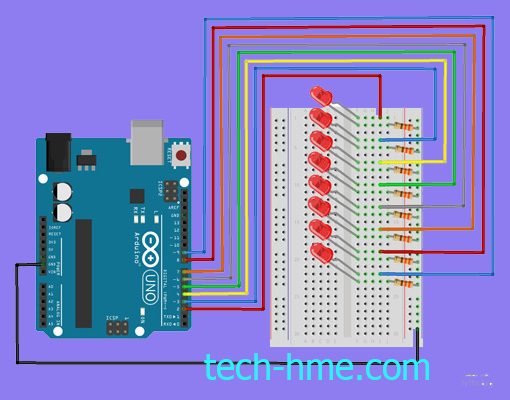# مصابيح LED متعددة باستخدام اردوينو

####```int ledPins[] = {4, 5, 6, 7, 8, 9};

void setup()
{
//create a local variable to store the index of whcih pin we want to control
int index;

// For the for() loop below, these are the three statements:

//   1. index = 0;    Before starting, make index = 0.
//   2. index <= 5;   If index is less or equal to 5, run the following code
//   3. index++   Putting "++" after a variable means "add one to it".

// When the test in statement 2 is finally false, the sketch
// will continue.

// This for() loop will make index = 0, then run the pinMode()
// statement within the brackets. It will then do the same thing
// for index = 2, index = 3, etc. all the way to index = 5.

for (index = 0; index <= 5; index++)
{
pinMode(ledPins[index], OUTPUT);
}
}

void loop()
{

// Light up all the LEDs in turn
oneAfterAnotherNoLoop();

// Same as oneAfterAnotherNoLoop, but less typing
//oneAfterAnotherLoop();

// Turn on one LED at a time, scrolling down the line
//oneOnAtATime();

// Light the LEDs middle to the edges
//pingPong();

// Chase lights like you see on signs
//marquee();

//randomLED();
}

/*
oneAfterAnotherNoLoop()
This function will light one LED, delay for delayTime, then light
the next LED, and repeat until all the LEDs are on. It will then
turn them off in the reverse order.
*/

void oneAfterAnotherNoLoop()
{
// time (milliseconds) to pause between LEDs
int delayTime = 100;

// turn all the LEDs on:

digitalWrite(ledPins, HIGH);  //Turns on LED #0 (pin 4)
delay(delayTime);                //wait delayTime milliseconds
digitalWrite(ledPins, HIGH);  //Turns on LED #1 (pin 5)
delay(delayTime);                //wait delayTime milliseconds
digitalWrite(ledPins, HIGH);  //Turns on LED #2 (pin 6)
delay(delayTime);                //wait delayTime milliseconds
digitalWrite(ledPins, HIGH);  //Turns on LED #3 (pin 7)
delay(delayTime);                //wait delayTime milliseconds
digitalWrite(ledPins, HIGH);  //Turns on LED #4 (pin 8)
delay(delayTime);                //wait delayTime milliseconds
digitalWrite(ledPins, HIGH);  //Turns on LED #5 (pin 9)
delay(delayTime);                //wait delayTime milliseconds

// turn all the LEDs off:

digitalWrite(ledPins, LOW);   //Turn off LED #5 (pin 9)
delay(delayTime);                //wait delayTime milliseconds
digitalWrite(ledPins, LOW);   //Turn off LED #4 (pin 8)
delay(delayTime);                //wait delayTime milliseconds
digitalWrite(ledPins, LOW);   //Turn off LED #3 (pin 7)
delay(delayTime);                //wait delayTime milliseconds
digitalWrite(ledPins, LOW);   //Turn off LED #2 (pin 6)
delay(delayTime);                //wait delayTime milliseconds
digitalWrite(ledPins, LOW);   //Turn off LED #1 (pin 5)
delay(delayTime);                //wait delayTime milliseconds
digitalWrite(ledPins, LOW);   //Turn off LED #0 (pin 4)
delay(delayTime);                //wait delayTime milliseconds
}

/*
oneAfterAnotherLoop()

This function does exactly the same thing as oneAfterAnotherNoLoop(),
but it takes advantage of for() loops and the array to do it with
much less typing.
*/

void oneAfterAnotherLoop()
{
int index;
int delayTime = 100; // milliseconds to pause between LEDs
// make this smaller for faster switching

// Turn all the LEDs on:

// This for() loop will step index from 0 to 5
// (putting "++" after a variable means add one to it)
// and will then use digitalWrite() to turn that LED on.

for (index = 0; index <= 5; index++)
{
digitalWrite(ledPins[index], HIGH);
delay(delayTime);
}

// Turn all the LEDs off:

// This for() loop will step index from 5 to 0
// (putting "--" after a variable means subtract one from it)
// and will then use digitalWrite() to turn that LED off.

for (index = 5; index >= 0; index--)
{
digitalWrite(ledPins[index], LOW);
delay(delayTime);
}
}

/*
oneOnAtATime()

This function will step through the LEDs,
lighting only one at at time.
*/

void oneOnAtATime()
{
int index;
int delayTime = 100; // milliseconds to pause between LEDs
// make this smaller for faster switching

// step through the LEDs, from 0 to 5

for (index = 0; index <= 5; index++)
{
digitalWrite(ledPins[index], HIGH);  // turn LED on
delay(delayTime);                    // pause to slow down
digitalWrite(ledPins[index], LOW);   // turn LED off
}
}

/*
pingPong()

This function will step through the LEDs,
lighting one at at time in both directions.
*/

void pingPong()
{
int index;
int delayTime = 100; // milliseconds to pause between LEDs
// make this smaller for faster switching

// step through the LEDs, from 0 to 5

for (index = 0; index <= 5; index++)
{
digitalWrite(ledPins[index], HIGH);  // turn LED on
delay(delayTime);                    // pause to slow down
digitalWrite(ledPins[index], LOW);   // turn LED off
}

// step through the LEDs, from 5 to 0

for (index = 5; index >= 0; index--)
{
digitalWrite(ledPins[index], HIGH);  // turn LED on
delay(delayTime);                    // pause to slow down
digitalWrite(ledPins[index], LOW);   // turn LED off
}
}

/*
marquee()

This function will mimic "chase lights" like those around signs.
*/

void marquee()
{
int index;
int delayTime = 200; // milliseconds to pause between LEDs
// Make this smaller for faster switching

// Step through the first four LEDs
// (We'll light up one in the lower 3 and one in the upper 3)

for (index = 0; index <= 2; index++) // Step from 0 to 3
{
digitalWrite(ledPins[index], HIGH);    // Turn a LED on
digitalWrite(ledPins[index + 3], HIGH); // Skip four, and turn that LED on
delay(delayTime);                      // Pause to slow down the sequence
digitalWrite(ledPins[index], LOW);     // Turn the LED off
digitalWrite(ledPins[index + 3], LOW); // Skip four, and turn that LED off
}
}

/*
randomLED()

This function will turn on random LEDs. Can you modify it so it
also lights them for random times?
*/

void randomLED()
{
int index;
int delayTime;

// The random() function will return a semi-random number each
// time it is called. See http://arduino.cc/en/Reference/Random
// for tips on how to make random() even more random.

index = random(5);    // pick a random number between 0 and 5
delayTime = 100;

digitalWrite(ledPins[index], HIGH);  // turn LED on
delay(delayTime);                    // pause to slow down
digitalWrite(ledPins[index], LOW);   // turn LED off
}```